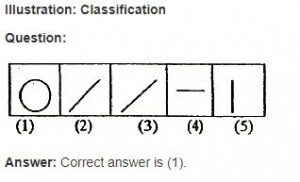## Visual Reasoning Classification

### Suggested Lessons

classification

In classification the problem figures themselves are the answer figures. Out of the five given figures four are similar in a certain way i.e. they exhibit a relation. One figure is not like the other four. You have to identify the “odd man out”.Explanation: In thefigures given. above,of the five figures four are straight lines whereas one is a circle. Thus the circle is the “odd man out “.